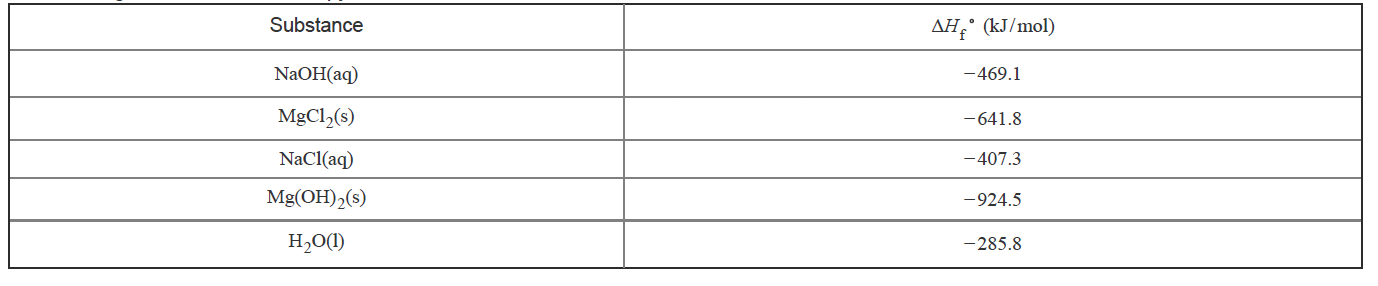# Problem: Determine the enthalpy for this reaction:MgCl2(s) + 2 NaOH(aq) → Mg(OH)2(s) + 2 NaCl(aq)Express your answer in kilojoules per mole to one decimal place.Enthalpy ΔH is a measure of the energy content of a system at constant pressure. Chemical reactions involve changes in enthalpy, ΔH, which can be measured and calculated: ΔH°rxn = ΣmΔH°f, products − ΣnΔH°f, reactantswhere the subscript "rxn" is for "enthalpy of reaction" and "f" is for "enthalpy of formation" and mm and n represent the appropriate stoichiometric coefficients for each substance.The following table lists some enthalpy of formation values for selected substances.

###### FREE Expert Solution
92% (38 ratings)View Complete Written Solution
###### Problem Details

Determine the enthalpy for this reaction:

MgCl2(s) + 2 NaOH(aq) → Mg(OH)2(s) + 2 NaCl(aq)

Enthalpy ΔH is a measure of the energy content of a system at constant pressure. Chemical reactions involve changes in enthalpy, ΔH, which can be measured and calculated:

ΔH°rxn = ΣmΔH°f, products − ΣnΔH°f, reactants

where the subscript "rxn" is for "enthalpy of reaction" and "f" is for "enthalpy of formation" and mm and n represent the appropriate stoichiometric coefficients for each substance.

The following table lists some enthalpy of formation values for selected substances.